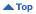Educational
HomeEducationalThe Sun and Solar ActivityGeneral InfoTemperature, Energy and Spectral Radiation Sunday, May 31 2020 07:08 UT

# Section Information

The Sun and Solar Activity

# Temperature, Energy and Spectral Radiation

As matter is heated, its component particles increase in energy and move faster. Electromagnetic energy is radiated over a wide bandwidth. As the temperature increases so does the frequency of the most prolific radiation. Here are some fundamental physical formulae that enable calculation of these parameters.

At an absolute temperature of T Kelvin, the thermal energy (E) of molecules is given by:

E = k T (joules) {approximate}

where k = 1.38 x 10-23 J /molecule/K is Boltzmann's constant. It is sometimes more convenient to express the energy in electron volts (eV) which is a much smaller unit than a joule (J), and is the energy of an electron after it has been accelerated through a potential difference of one volt. Thus, the energy in electron volts is given by:

V = E / qe (eV)

where qe = 1.6 x 10-19 Coulombs is the charge on an electron.

In a plasma, where some electrons are free to wander around on their own, we can define a thermal velocity (ve) by equating the above energy to the electron kinetic energy:

ve = √ ( 2 E / me ) (m/s)

where me = 9.11 x 10-31 kg is the mass of an electron

The wavelength of maximum radiation (lm) is given by the Wien Displacement law:

lm = 0.0029 / T (m)

These quantities are tabulated as a function of temperature in the table below. The spectral classification of the radiation is also indicated. Note that normal thermal radiation is broadband. However, if an atom suffers a collision with a free electron, a bound electron may be ejected from the atom. Another electron may fall back into the bound position and single frequency spectral line radiation will be emitted.

Solar radiation occurs at all wavelengths listed below. From the photosphere, at a temperature of 6000 K, both infrared and visible radiation are emitted. High in the Sun's atmosphere, the temperature increases, and UV and X- rays are emitted. Some of the radiation is thermal (broadband) and some is line (spectral) radiation. Solar images at different wavelengths depict different altitudes and processes.

Temperature (K) Energy (eV) RMS Velocity (km/s) Wavelength (nm) Spectrum (Class)
1000 0.1 174 2900.0 Infrared
3000 0.3 301 966.7 Infrared
7000 0.6 461 414.3 Visible
10000 0.9 550 290.0 Ultraviolet
30000 2.6 953 96.7 Extreme UV
70000 6.0 1456 41.4 Extreme UV
100000 8.6 1741 29.0 Extreme UV
300000 25.9 3015 9.7 Soft X-Ray
700000 60.4 4605 4.1 Soft X-Ray
1000000 86.3 5504 2.9 Soft X-Ray
3000000 258.8 9534 1.0 Soft X-Ray
7000000 603.8 14563 0.4 Soft X-Ray

Material prepared by John Kennewell